#PyTorch可视化理解卷积神经网络

1. 云栖社区>
2. 翻译小组>
3. 博客>
4. 正文

## PyTorch可视化理解卷积神经网络

【方向】 2019-01-20 12:05:29 浏览1996

如今，机器已经能够在理解、识别图像中的特征和对象等领域实现99％级别的准确率。生活中，我们每天都会运用到这一点，比如，智能手机拍照的时候能够识别脸部、在类似于谷歌搜图中搜索特定照片、从条形码扫描文本或扫描书籍等。造就机器能够获得在这些视觉方面取得优异性能可能是源于一种特定类型的神经网络——卷积神经网络（CNN）。如果你是一个深度学习爱好者，你可能早已听说过这种神经网络，并且可能已经使用一些深度学习框架比如caffe、TensorFlow、pytorch实现了一些图像分类器。然而，这仍然存在一个问题：数据是如何在人工神经网络传送以及计算机是如何从中学习的。为了从头开始获得清晰的视角，本文将通过对每一层进行可视化以深入理解卷积神经网络。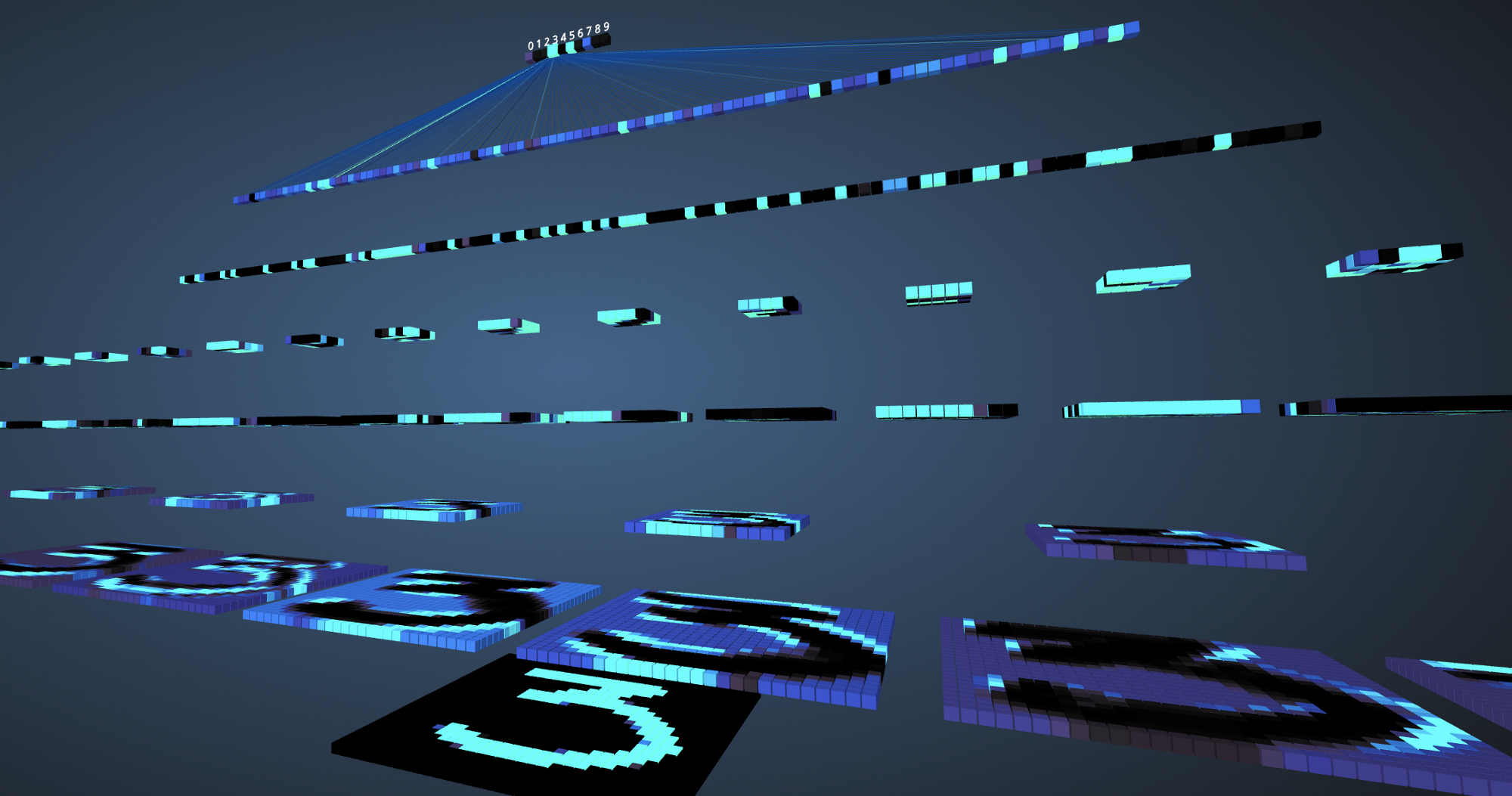## 卷积神经网络

在学习卷积神经网络之前，首先要了解神经网络的工作原理。神经网络是模仿人类大脑来解决复杂问题并在给定数据中找到模式的一种方法。在过去几年中，这些神经网络算法已经超越了许多传统的机器学习和计算机视觉算法。“神经网络”是由几层或多层组成，不同层中具有多个神经元。每个神经网络都有一个输入和输出层，根据问题的复杂性增加隐藏层的个数。一旦将数据送入网络中，神经元就会学习并进行模式识别。一旦神经网络模型被训练好后，模型就能够预测测试数据。
另一方面，CNN是一种特殊类型的神经网络，它在图像领域中表现得非常好。该网络是由YanLeCunn在1998年提出的，被应用于数字手写体识别任务中。其它应用领域包括语音识别、图像分割和文本处理等。在CNN被发明之前，多层感知机（MLP）被用于构建图像分类器。图像分类任务是指从多波段（彩色、黑白）光栅图像中提取信息类的任务。MLP需要更多的时间和空间来查找图片中的信息，因为每个输入元素都与下一层中的每个神经元连接。而CNN通过使用称为局部连接的概念避免这些，将每个神经元连接到输入矩阵的局部区域。这通过允许网络的不同部分专门处理诸如纹理或重复模式的高级特征来最小化参数的数量。下面通过比较说明上述这一点。

## 比较MLP和CNN

因为输入图像的大小为`28x28=784`（MNIST数据集），MLP的输入层神经元总数将为784。网络预测给定输入图像中的数字，输出数字范围是0-9。在输出层，一般返回的是类别分数，比如说给定输入是数字“3”的图像，那么在输出层中，相应的神经元“3”与其它神经元相比具有更高的类别分数。这里又会出现一个问题，模型需要包含多少个隐藏层，每层应该包含多少神经元？这些都是需要人为设置的，下面是一个构建MLP模型的例子：

``````Num_classes = 10
Model = Sequntial()
Model.add(Dense(512, activation=’relu’, input_shape=(784, )))

上面的代码片段是使用Keras框架实现（暂时忽略语法错误），该代码表明第一个隐藏层中有512个神经元，连接到维度为784的输入层。隐藏层后面加一个dropout层，丢弃比例设置为0.2，该操作在一定程度上克服过拟合的问题。之后再次添加第二个隐藏层，也具有512谷歌神经元，然后再添加一个dropout层。最后，使用包含10个类的输出层完成模型构建。其输出的向量中具有最大值的该类将是模型的预测结果。
这种多层感知器的一个缺点是层与层之间完全连接，这导致模型需要花费更多的训练时间和参数空间。并且，MLP只接受向量作为输入。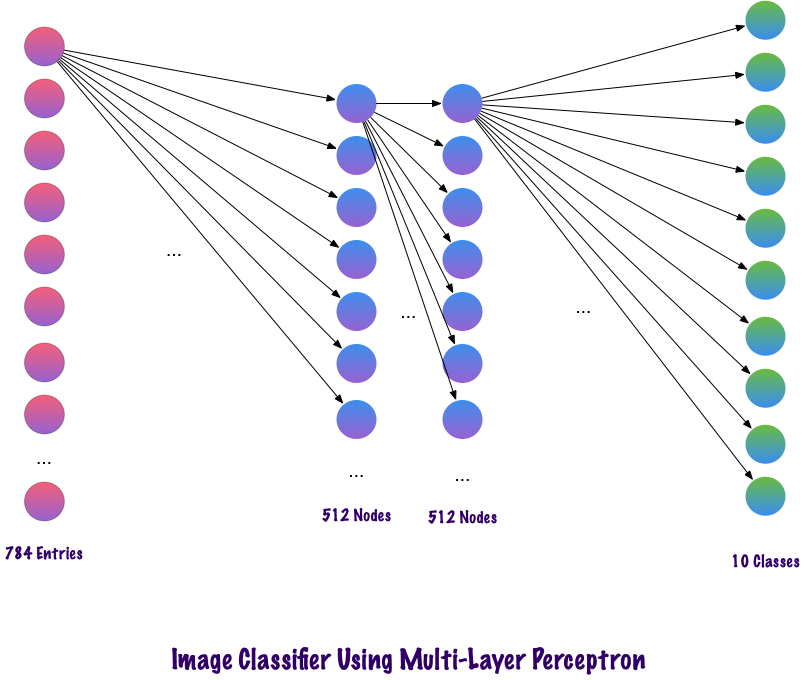卷积使用稀疏连接的层，并且其输入可以是矩阵，优于MLP。输入特征连接到局部编码节点。在MLP中，每个节点都有能力影响整个网络。而CNN将图像分解为区域（像素的小局部区域），每个隐藏节点与输出层相关，输出层将接收的数据进行组合以查找相应的模式。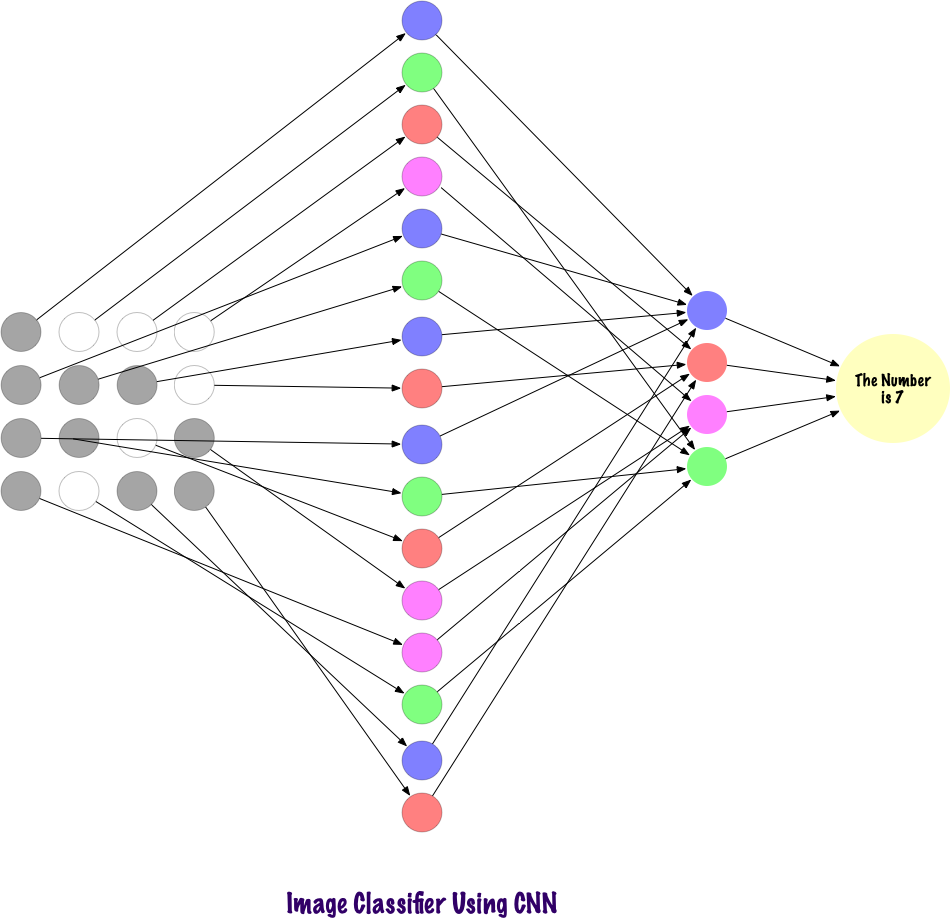## 计算机如何查看输入的图像？

看着图片并解释其含义，这对于人类来说很简单的一件事情。我们生活在世界上，我们使用自己的主要感觉器官（即眼睛）拍摄环境快照，然后将其传递到视网膜。这一切看起来都很有趣。现在让我们想象一台计算机也在做同样的事情。

``````# Load the libraries
import torch
import numpy as np

from torchvision import datasets
import torchvision.transforms as transforms

# Set the parameters
num_workers = 0
batch_size = 20

# Converting the Images to tensors using Transforms
transform = transforms.ToTensor()

train_data = datasets.MNIST(root='data', train=True,
test_data = datasets.MNIST(root='data', train=False,

num_workers=num_workers)
num_workers=num_workers)

import matplotlib.pyplot as plt
%matplotlib inline

images, labels = dataiter.next()
images = images.numpy()

# Peeking into dataset
fig = plt.figure(figsize=(25, 4))
for image in np.arange(20):
ax = fig.add_subplot(2, 20/2, image+1, xticks=[], yticks=[])
ax.imshow(np.squeeze(images[image]), cmap='gray')
ax.set_title(str(labels[image].item()))``````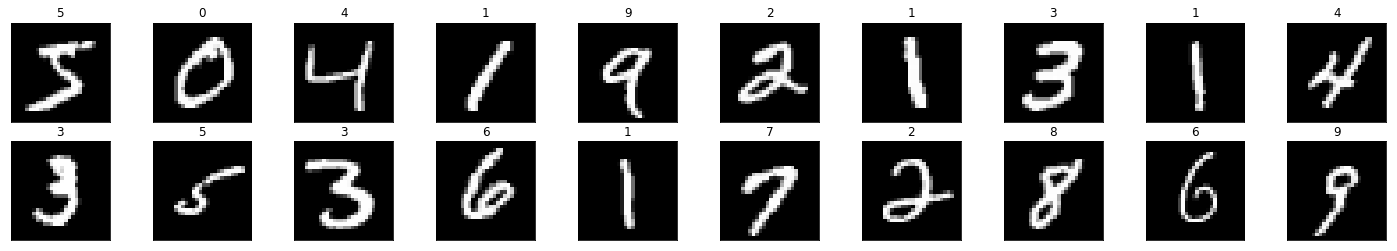``````img = np.squeeze(images)

fig = plt.figure(figsize = (12,12))
ax.imshow(img, cmap='gray')
width, height = img.shape
thresh = img.max()/2.5
for x in range(width):
for y in range(height):
val = round(img[x][y],2) if img[x][y] !=0 else 0
ax.annotate(str(val), xy=(y,x),
color='white' if img[x][y]<thresh else 'black')``````

上述代码将数字'3'图像分解为像素。在一组手写数字中，随机选择“3”。并且将实际像素值（0-255 ）标准化，并将它们限制在0到1的范围内。归一化的操作能够加快模型训练收敛速度。

## 构建过滤器

过滤器，顾名思义，就是过滤信息。在使用CNN处理图像时，过滤像素信息。为什么需要过滤呢，计算机应该经历理解图像的学习过程，这与孩子学习过程非常相似，但学习时间会少的多。简而言之，它通过从头学习，然后从输入层传到输出层。因此，网络必须首先知道图像中的所有原始部分，即边缘、轮廓和其它低级特征。检测到这些低级特征之后，传递给后面更深的隐藏层，提取更高级、更抽象的特征。过滤器提供了一种提取用户需要的信息的方式，而不是盲目地传递数据，因为计算机不会理解图像的结构。在初始情况下，可以通过考虑特定过滤器来提取低级特征，这里的滤波器也是一组像素值，类似于图像。可以理解为连接卷积神经网络中的权重。这些权重或滤波器与输入相乘以得到中间图像，描绘了计算机对图像的部分理解。之后，这些中间层输出将与多个过滤器相乘以扩展其视图。然后提取到一些抽象的信息，比如人脸等。
就“过滤”而言，我们有很多类型的过滤器。比如模糊滤镜、锐化滤镜、变亮、变暗、边缘检测等滤镜。

``````Import matplotlib.pyplot as plt
Import matplotib.image as mpimg
Import cv2
Import numpy as np

Plt.imshow(image)````````````# 转换为灰度图
gray = cv2.cvtColor(image, cv2.COLOR_RB2GRAY)

# 定义sobel过滤器
sobel = np.array([-1, -2, -1],
[0, 0, 0],
[1, 2, 1]))
# 应用sobel过滤器
Filtered_image = cv2.filter2D(gray, -1, sobel_y)
# 画图
Plt.imshow(filtered_image, cmp=’gray’)``````## 完整的CNN结构

到目前为止，已经看到了如何使用滤镜从图像中提取特征。现在要完成整个卷积神经网络，cnn使用的层是：

• 1.卷积层（Convolutional layer）
• 2.池层(Pooling layer)
• 3.全连接层(fully connected layer)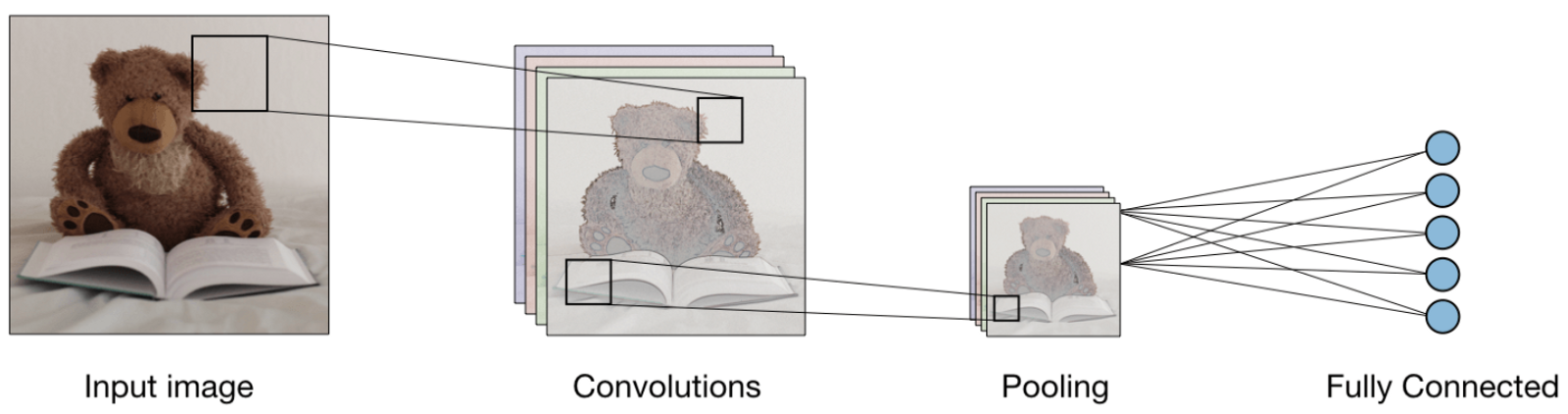* 卷积层（CONV）——使用过滤器执行卷积操作。因为它扫描输入图像的尺寸。它的超参数包括滤波器大小，可以是2x2、3x3、4x4、5x5（或其它）和步长S。结果输出O称为特征映射或激活映射，具有使用输入层计算的所有特征和过滤器。下面描绘了应用卷积的工作过程：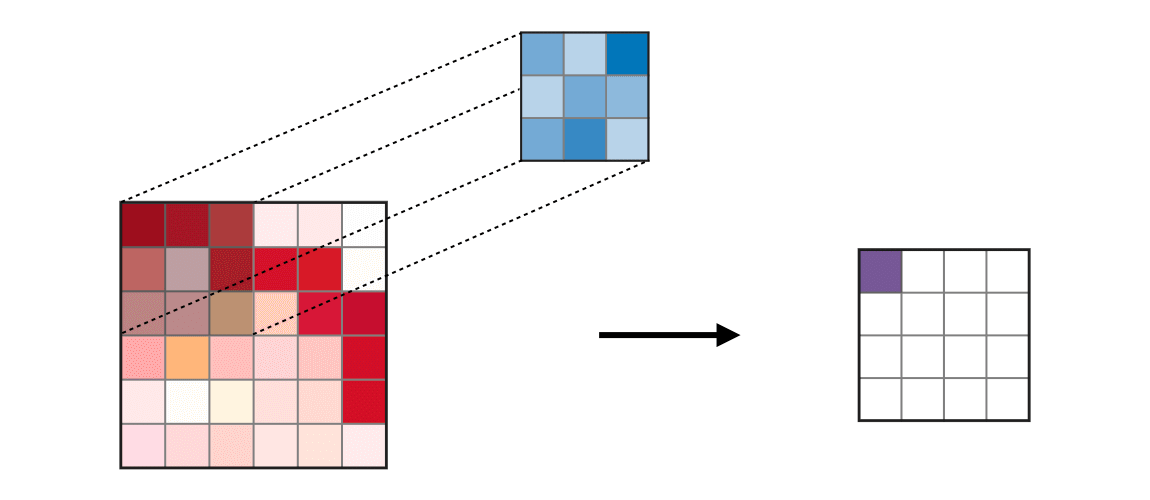• 池化层（POOL）——用于特征的下采样，通常在卷积层之后应用。池化处理方式有多种类型，常见的是最大池化（max pooling）和平均池化（ave pooling），分别采用特征的最大值和平均值。下面描述了池化的工作过程：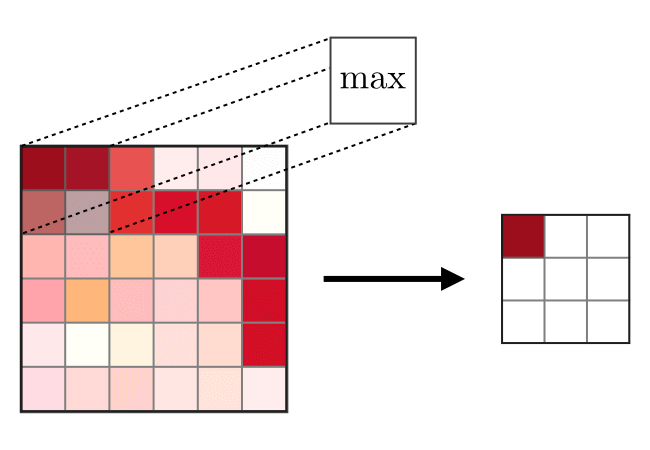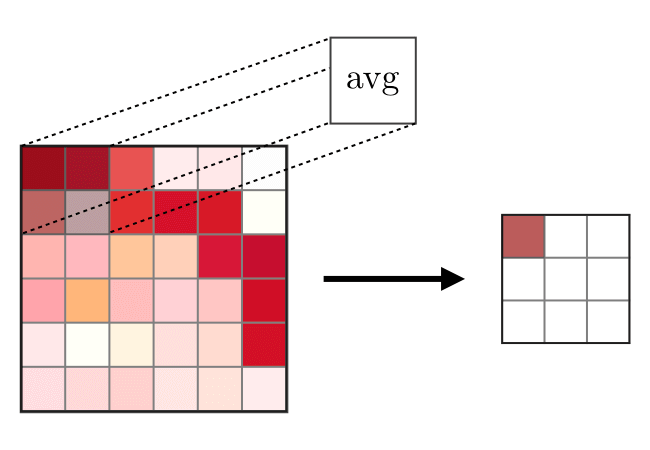•  全连接层（FC）——在展开的特征上进行操作，其中每个输入连接到所有的神经元，通常在网络末端用于将隐藏层连接到输出层，下图展示全连接层的工作过程：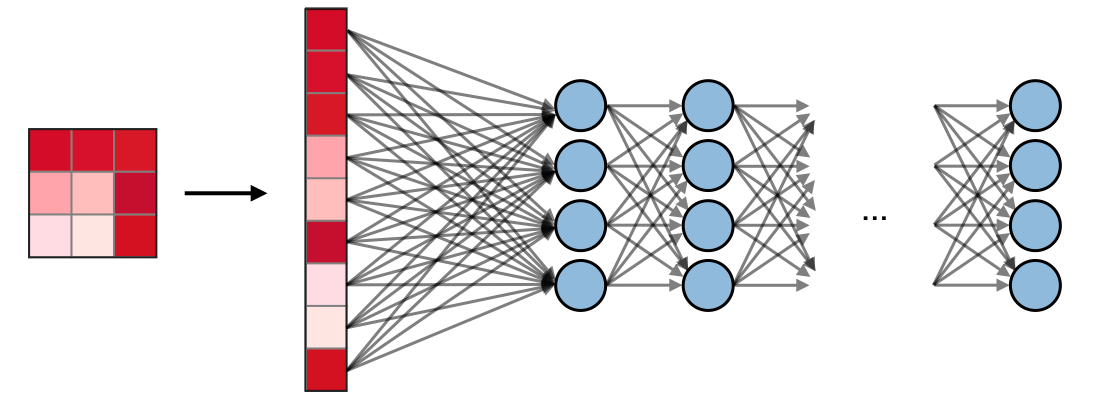## 在PyTorch中可视化CNN

``````import cv2
import matplotlib.pyplot as plt
%matplotlib inline

img_path = 'dog.jpg'

gray_img = cv2.cvtColor(bgr_img, cv2.COLOR_BGR2GRAY)

# Normalise
gray_img = gray_img.astype("float32")/255

plt.imshow(gray_img, cmap='gray')
plt.show()````````````import numpy as np

filter_vals = np.array([
[-1, -1, 1, 1],
[-1, -1, 1, 1],
[-1, -1, 1, 1],
[-1, -1, 1, 1]
])

print('Filter shape: ', filter_vals.shape)

# Defining the Filters
filter_1 = filter_vals
filter_2 = -filter_1
filter_3 = filter_1.T
filter_4 = -filter_3
filters = np.array([filter_1, filter_2, filter_3, filter_4])

# Check the Filters
fig = plt.figure(figsize=(10, 5))
for i in range(4):
ax = fig.add_subplot(1, 4, i+1, xticks=[], yticks=[])
ax.imshow(filters[i], cmap='gray')
ax.set_title('Filter %s' % str(i+1))
width, height = filters[i].shape
for x in range(width):
for y in range(height):
ax.annotate(str(filters[i][x][y]), xy=(y,x),
color='white' if filters[i][x][y]<0 else 'black')
``````

``````import torch
import torch.nn as nn
import torch.nn.functional as F

class Net(nn.Module):

def __init__(self, weight):
super(Net, self).__init__()
# initializes the weights of the convolutional layer to be the weights of the 4 defined filters
k_height, k_width = weight.shape[2:]
# assumes there are 4 grayscale filters
self.conv = nn.Conv2d(1, 4, kernel_size=(k_height, k_width), bias=False)
# initializes the weights of the convolutional layer
self.conv.weight = torch.nn.Parameter(weight)
# define a pooling layer
self.pool = nn.MaxPool2d(2, 2)

def forward(self, x):
# calculates the output of a convolutional layer
# pre- and post-activation
conv_x = self.conv(x)
activated_x = F.relu(conv_x)

# applies pooling layer
pooled_x = self.pool(activated_x)

# returns all layers
return conv_x, activated_x, pooled_x

# instantiate the model and set the weights
weight = torch.from_numpy(filters).unsqueeze(1).type(torch.FloatTensor)
model = Net(weight)

# print out the layer in the network
print(model)``````

Net(

(conv): Conv2d(1, 4, kernel_size=(4, 4), stride=(1, 1), bias=False)
(pool): MaxPool2d(kernel_size=2, stride=2, padding=0, dilation=1, ceil_mode=False)
)

``````def viz_layer(layer, n_filters= 4):
fig = plt.figure(figsize=(20, 20))

for i in range(n_filters):
ax = fig.add_subplot(1, n_filters, i+1)
ax.imshow(np.squeeze(layer[0,i].data.numpy()), cmap='gray')
ax.set_title('Output %s' % str(i+1))

fig = plt.figure(figsize=(12, 6))
fig.subplots_adjust(left=0, right=1.5, bottom=0.8, top=1, hspace=0.05, wspace=0.05)
for i in range(4):
ax = fig.add_subplot(1, 4, i+1, xticks=[], yticks=[])
ax.imshow(filters[i], cmap='gray')
ax.set_title('Filter %s' % str(i+1))

gray_img_tensor = torch.from_numpy(gray_img).unsqueeze(0).unsqueeze(1)``````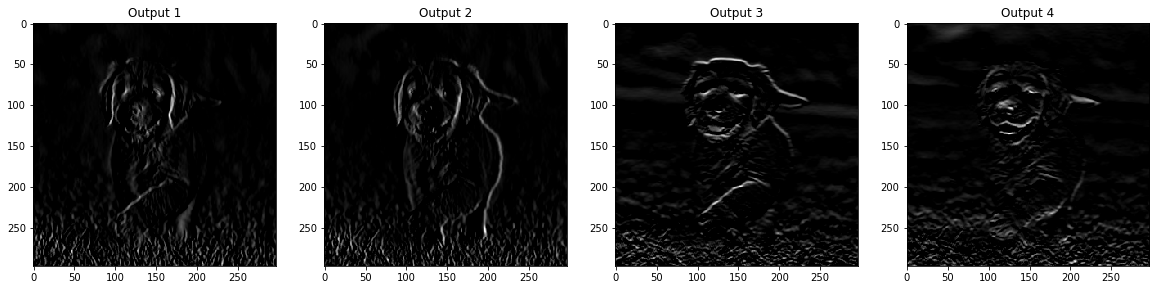## 作者信息

Vihar Kurama，机器学习